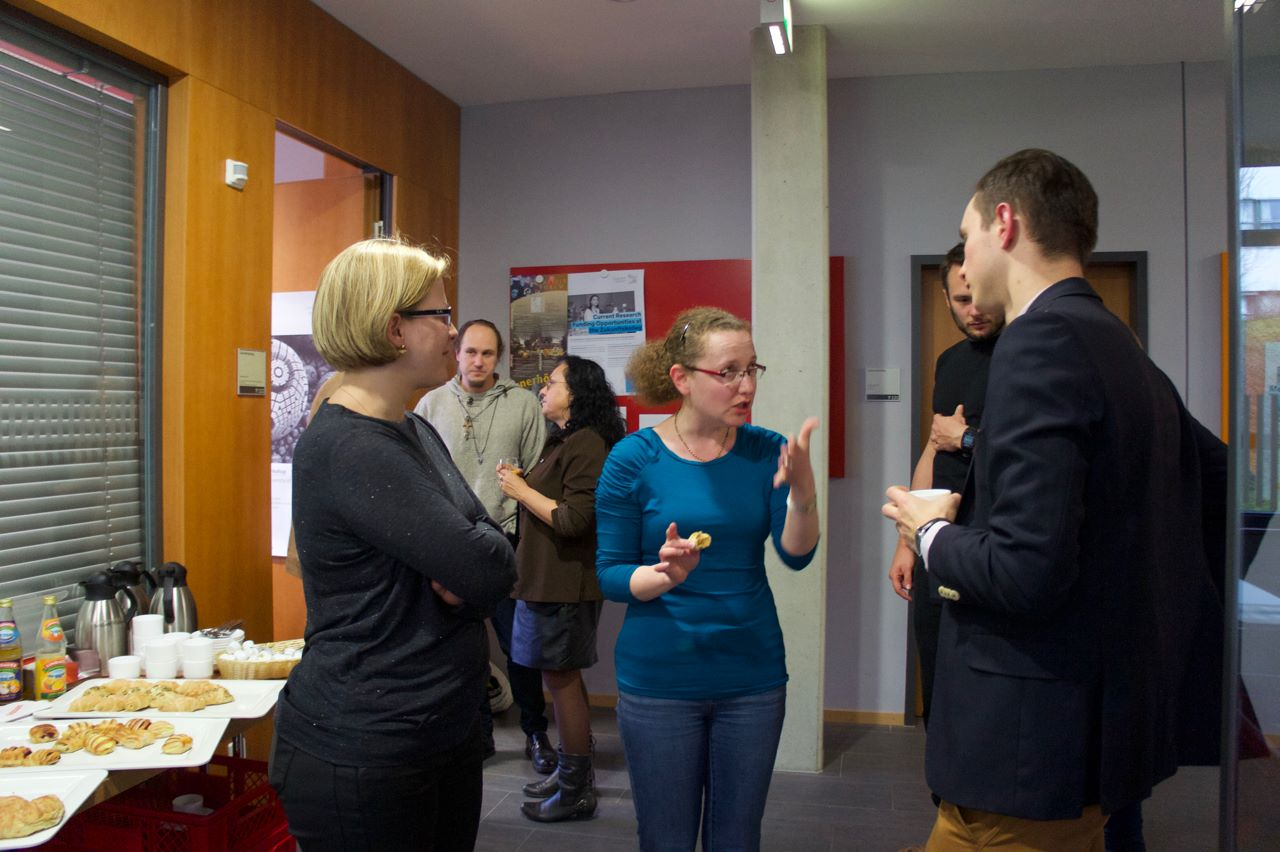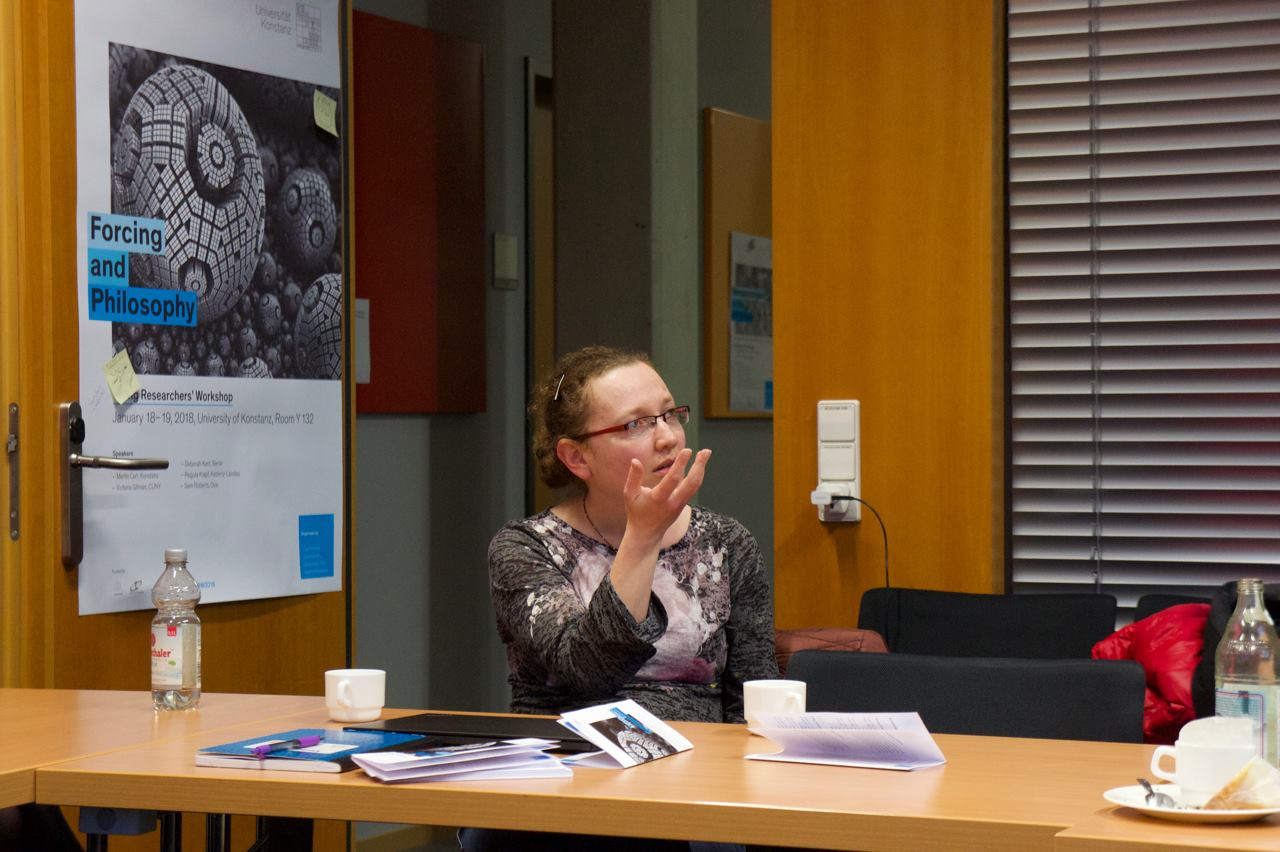The emerging zoo of second-order set theories

This is a talk at the Young Researchers' Workshop: Forcing and Philosophy, University of Konstanz, January 18, 2018.
SlidesIn set theory, classes are collections of sets which are too large" to be sets themselves. More formally, a class, in a model of first-order set theory, is a definable (with parameters) collection of sets. Classes play an important role in modern set theory. Class forcing can make global changes to a universe of set theory, such as making the ${\rm GCH}$ fail at all regular cardinals. Large cardinals imply the existence of class elementary embeddings $j:V\to M$ from the universe into a transitive class submodel. Inner model theory studies canonical inner models (transitive class submodels containing all the ordinals).

The restriction to the study of only the definable large collections of sets is a limitation of first-order set theory which prevents us from exploring some natural properties of set-theoretic universes. For instance, consider the long standing open question whether Reinhardt cardinals are consistent with ${\rm ZF}$, which has been revisited only a few days ago in this article. The Reinhardt cardinal is the critical point of an elementary embedding $j:V\to V$. It is not difficult to show that there cannot be a definable elementary $j:V\to V$ in a model of ${\rm ZF}$, so the open question is about the existence of an undefinable such embedding. Other recent examples of the use of general classes comes from the study of inner model reflection principles. Motivated by a question of Neil Barton, Barton, Caicedo, Fuchs, Hamkins, and Reitz recently introduced and studied the Inner Model Reflection Principle stating that every first-order formula reflects to a proper inner model . The statement of the principle cannot be expressed in first-order set theory because it requires quantifying over classes. Along similar lines, Friedman had previously introduced the Inner Model Hypothesis which states that if a first-order sentence holds in an outer model (extension universe) of an inner model, then it already holds in some inner model . For a long time it was not clear in what framework this principle could be formalized because it requires not only quantifying over classes but also referring to classes that are potentially outside the universe itself.

So how do we undertake a general study of classes? What is the framework in which we can have undefinable classes and where we can study the properties of classes in the same way we study sets? This framework is second-order set theory, formalized in a two-sorted logic with separate objects and quantifiers for sets and classes. Models of second-order set theory are triples $\mathscr V=\langle V,\in,\mathcal C\rangle$ where $\mathcal C$ is the collection of classes of $\mathscr V$. One of the weakest reasonable axiomatizations of second-order theory is the Gödel-Bernays set theory ${\rm GBC}$ whose axioms consist of the ${\rm ZFC}$ axioms for sets, extensionality, replacement, and existence of global well-order axioms for classes, together with a weak comprehension scheme stating that every first-order formula defines a class. If a universe of set theory has a definable global well-order, then it together with its definable classes is a model of ${\rm GBC}$. Indeed, ${\rm GBC}$ is equiconsistent with ${\rm ZFC}$ and has the same first-order consequences as ${\rm ZFC}$. If we just add to ${\rm GBC}$ comprehension for $\Sigma^1_1$-formulas (formulas with a single class existential quantifier), we get a much stronger theory with many desirable properties. The theory ${\rm GBC}$ + $\Sigma^1_1$-Comprehension implies that that any two meta-ordinals (class well-orders) are comparable, that we can iterate the $L$ construction along any meta-ordinal, that there is an iterated truth predicate along any meta-ordinal, that determinacy holds for open class games, and that the class forcing theorem holds.

A truth predicate is a class of Gödel codes of first-order formulas obeying Tarskian truth conditions. Tarski's Theorem on the undefinablity of truth implies that a truth predicate cannot be definable and therefore ${\rm GBC}$, because it can have models with only the definable classes, cannot imply the existence of such a class. Indeed, the existence of a truth predicate class implies $\text{Con}({\rm ZFC})$, $\text{Con}(\text{Con}({\rm ZFC}))$ and much more. ${\rm GBC}$ + $\Sigma^1_1$-Comprehension implies that there is a truth predicate for every structure $\langle V,\in, A\rangle$ for a class $A$. In particular, if $T_0$ is the truth predicate (for $\langle V,\in,A\rangle$), then we have a truth predicate $T_1$ for the structure $\langle V,\in, T_0,A\rangle$, that is we have truth for truth. How far can we iterate the truth operation? ${\rm GBC}$ + $\Sigma^1_1$-Comprehension implies that we get an iterated truth predicate along any meta-ordinal.

By analogy with games on $X^\omega$, for a set $X$, where the players take turns playing elements from $X$ for $\omega$-many steps, in the second-order context we can consider games on ${\rm ORD}^\omega$. It turns out ${\rm GBC}$ + $\Sigma^1_1$-Comprehension implies determinacy for all such open class games .

The strength of the forcing construction comes from the Forcing Theorem which states that the forcing relation (for a fixed first-order formula) is definable. The analogue of the Forcing Theorem for class partial orders says that the forcing relation (for a fixed first-order formula) is a class. The Class Forcing Theorem can fail in a model of ${\rm GBC}$ because there are class forcing notions from whose forcing relation for atomic formulas we can define a truth predicate. But ${\rm GBC}$ + $\Sigma^1_1$-Comprehension implies the Class Forcing Theorem.

Surprisingly, in ${\rm GBC}$ + $\Sigma^1_1$-Comprehension, we can even formalize Friedman's Inner Model Hypothesis because the properties of outer models can be expressed via a strong logic, called $V$-logic, whose proof system is expressible in this theory .

Indeed, it turns out that most of these principles are implied by a weaker natural theory ${\rm GBC}$ + ${\rm ETR}$ elementary transfinite recursion. The principle ${\rm ETR}$, which is an analogue of the Recursion Theorem in first-order set theory, states that every first-order definable recursion along a meta-ordinal has a solution. The principle ${\rm ETR}$ implies over ${\rm GBC}$ that we can iterate the $L$ construction along any meta-ordinal. Over ${\rm GBC}$, the principle ${\rm ETR}$ is equivalent to determinacy for clopen class games and to the existence of an iterated truth predicate along any meta-ordinal . The Class Forcing Theorem is equivalent over ${\rm GBC}$, to the principle $\rm {ETR}_{\rm {ORD}}$, stating that we can perform recursions along ${\rm ORD}$ . The amount of available ${\rm ETR}$ gives a natural hierarchy of second-order set theories above ${\rm GBC}$ with ${\rm ETR}_\omega$ already implying the existence of a truth predicate.

The only principle we have considered so far which is known to be stronger than ${\rm ETR}$ is open determinacy, a result due to Sato . Hamkins and Woodin showed recently that open determinacy implies that forcing does not add meta-ordinals, a natural analogue to the statement that forcing does not add ordinals (personal communication).

Of course, the amount of available comprehension itself gives a hierarchy of second-order set theories culminating with the Kelley-Morse set theory ${\rm KM}$, which consists of ${\rm GBC}$ together with the full comprehension scheme for all second-order formulas. Beyond ${\rm KM}$ are theories which include choice principles for classes, such as the choice scheme and the dependent choice scheme. These theories have the advantage of bi-interpretability with extensions of the well-understood first-order set theory ${\rm ZFC}^-_I$ (${\rm ZFC}$ without powerset and with the existence of the largest cardinal which is inaccessible). An even stronger principle, which endows classes with more set-like properties, is the existence of a canonically definable well-order of the classes. The existence of a definable well-ordering on classes makes it possible, for instance, to carry out the Boolean valued model forcing construction for class forcing notions (work in progress with Carolin Antos and Sy-David Friedman).

Are there natural second-order set-theoretic principles between ${\rm GBC}$ + $\Sigma^1_1$-comprehension and ${\rm KM}?$ What natural principles lie beyond ${\rm KM}$ together with the choice scheme and the dependent choice scheme?

References

1. N. Barton, A. E. Caicedo, G. Fuchs, J. D. Hamkins, and J. Reitz, “Inner-model reflection principles,” ArXiv e-prints, 2017. Available at: http://jdh.hamkins.org/inner-model-reflection-principles
2. S.-D. Friedman, “Internal consistency and the inner model hypothesis,” Bull. Symbolic Logic, vol. 12, no. 4, pp. 591–600, 2006. Available at: http://projecteuclid.org/getRecord?id=euclid.bsl/1164056808
3. V. Gitman and J. D. Hamkins, “Open determinacy for class games,” in Foundations of mathematics, vol. 690, Amer. Math. Soc., Providence, RI, 2017, pp. 121–143.
4. N. Barton, C. Antos, and S.-D. Friedman, “Universism and extensions of V,” ArXiv e-prints.
5. V. Gitman, J. D. Hamkins, P. Holy, P. Schlicht, and K. Williams, “The exact strength of the class forcing theorem,” Submitted.
6. K. Sato, “Inductive dichotomy: separation of open and clopen class determinacies.”